#Wolfram 语言：快速编程入门# 数值视频

In:=1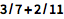`3/7 + 2/11`
Out=1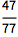JAVA 程序员备注：

Python 程序员备注：

In:=2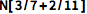`N[3/7+2/11]`
Out=2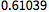Wolfram 语言可以处理任何精度的数值：

In:=1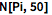`N[Pi,50]`
Out=1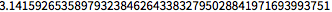` 以数值明确指明精度：

In:=2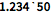`1.234`50`
Out=2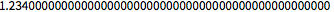JAVA 程序员备注：

Java 代码对于任意精度整数和小数必须明确使用 BigIntegerBigDecimal 类型.

Python 程序员备注：

Wolfram 语言中的数字可以像 Python 整数可变为任意大小. Floats 在 Python 中需要导入第三方库 mpmath.

In:=1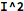`I^2`
Out=1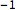JAVA 程序员备注：

Java 没有虚数 i 的内置显示. 在 Java 中进行复数计算，必须导入或创建程序包.

Python 程序员备注：

Wolfram 语言提供了数量不同的格式化形式，例如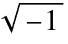用于虚数，并且与其他常数类似，来最大化明确性. 另外，无论数字是否为实数或复数，Wolfram 语言会在执行时候自动进行推论. Python 默认使用符号 j 来表示一个虚数，且在通用运算符中并不会一定会被运用.

In:=1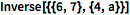`Inverse[{{6,7},{4,a}}]`
Out=1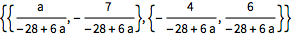SparseArray 给出稀疏数组.

JAVA 程序员备注：

Python 程序员备注：